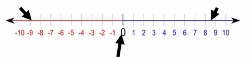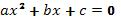# InequalitiesTo score 700+ on the GMAT, test takers must develop a strategy to answer the Quant questions in 2 minutes and Verbal questions in 1 minute & 20 seconds. Data sufficiency questions can be solved well within the 2-minute mark, most likely in 1 minute and 30 seconds if the conditions and question are rephrased. Not all questions will be required to be rephrased but there are certain conditions where this technique is extremely useful, especially when concepts in Ratio & Proportion, Equations, Inequalities & Divisibility are tested.EquationsThis is the most common question type where rephrasing the conditions might be useful, especially in quadratic equations, and questions that require finding roots. Let us look into a quadratic equation question typeFor the following equations with positive roots, the value of k is greater than one1) 3x^2 +5x + 2k = 02) (x+1)(3x+2) + 2k - 2 = 01) Statement (1) ALONE is sufficient, but statement (2) alone is not sufficient to answer the question asked.2) Statement (2) ALONE is sufficient, but statement (1) alone is not sufficient to answer the question asked.3) BOTH... Categories : Absolute Value, InequalitiesGood news, test takers! You can pass the GMAT! Most of the questions are not that difficult. The difficulty in the test is in taking a mid-level question, (5x2 = 10) and rephrasing it in a more difficult way. (5 men walk into a bar. The bartender gives each man a beer for each of their hands. How many beers does the bartender distribute all together?) There is one exception to the rule, though: Absolute value inequalities.It’s a little tough, but not impossible to figure out absolute value inequalities! We’ll start with absolute value expression’s basic definitions, mathematically and conceptually.  Then we’ll use that basic understanding to solve equations and inequalities including absolute value expressions. Finally, we’ll deal with a concept-based application of the absolute value inequalities on the GMAT. Let’s go! Absolute positive - Everybody knows that |9|=9 and|-9|=9.  What’s so special about those slanted lines? How and why does it neutralize a number?  Those bars are the measure of the distance of the number within the bars and zero. -9 and 9 are both 9 units away from 0. Picture it!... Categories : Inequalities Quadratic Inequalities is an important and often misunderstood topic in GMAT. But before we start, let us recall what a quadratic equation is and how is it represented.The standard form of a quadratic equation is:And the roots of this standard equation are:where b2 - 4ac  is known as a Discriminant, ?.This standard quadratic equation becomes an inequality if it is represented as(or > 0)The first step in solving a quadratic inequality problem...

Get F1GMAT's Newsletters (Best in the Industry)

• Ranking Analysis
• Post-MBA Salary Trends
• Post-MBA Job Function & Industry Analysis
• Post-MBA City Review
• MBA Application Essay Tips
• School Specific Essay Tips
• GMAT Preparation Tips